1. Which is a solution for the equation 2x - 4y = 12? (0,3) (0,-3) (-6,0) (2,2) 2. Which is a solution for the equation x - y = 6? (0,6) (7,-1) (0,-6) (8,-2) 3. For the equation x - 4y = 10, find the value of y if x = 2. 2 -2 3 0 4. Which is a solution for the equation y = 3x - 1? (3,0) (2,7) (0,1) (0,-1) 5. Which represents the graph of the equation 2x - y = 8?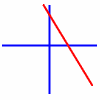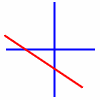6. Which represents the graph of the equation x + 2y = 10?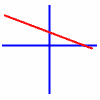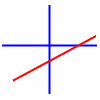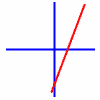7. Which represents the graph of the equation y = x/3?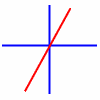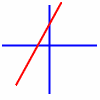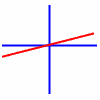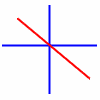8. Which represents the graph of the equation y = 2x - 2?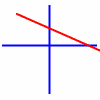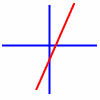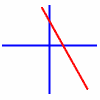9. The point (4,-5) is in what quadrant? I II III IV 10. The x intercept of the equation 3x + 4y = 12 is (4,0) (0,4) (3,0) (0,3)